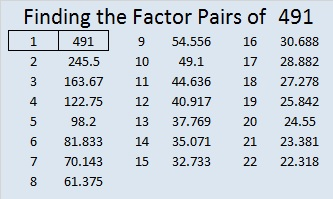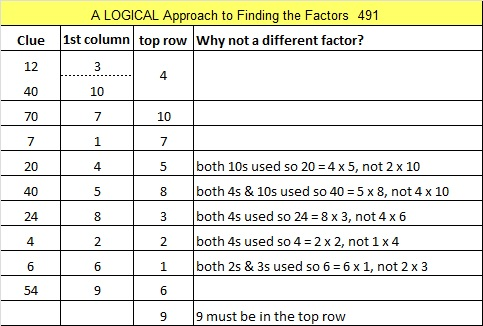491 and Level 4

491 is the sum of consecutive primes two different ways. Check the comments to see if any of my readers list what those consecutive primes are.Print the puzzles or type the solution on this excel file: 10 Factors 2015-05-11

—————————————————————————————————

• 491 is a prime number.
• Prime factorization: 491 is prime.
• The exponent of prime number 491 is 1. Adding 1 to that exponent we get (1 + 1) = 2. Therefore 491 has exactly 2 factors.
• Factors of 491: 1, 491
• Factor pairs: 491 = 1 x 491
• 491 has no square factors that allow its square root to be simplified. √491 ≈ 22.1585198How do we know that 491 is a prime number? If 491 were not a prime number, then it would be divisible by at least one prime number less than or equal to √491 ≈ 22.159. Since 491 cannot be divided evenly by 2, 3, 5, 7, 11, 13, 17, or 19, we know that 491 is a prime number.

—————————————————————————————————